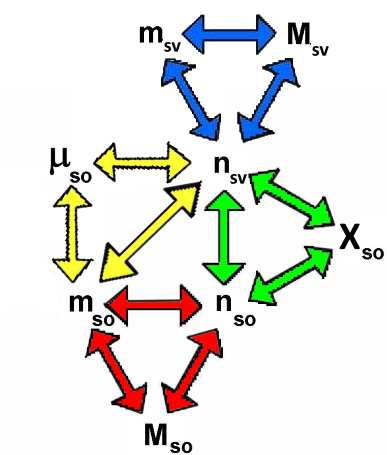Search:

# Two concentrations: Molality and mole fraction

## Molality ($\mu$)

The molality of a solute is the ratio of the number of moles of solute per mass (in $g$) of the solvent, multiplied by $1000$ or: The molality of the solute is the ratio of the number of moles of solute by the mass ( in kg) of solvent,

Molality of a solute: $\mu_{so}$ $=$ $\frac{n_{so}\cdot 1000}{m_{sv}}$

For example, $\mu_{so}$ $=$ $2.4$ means that $1000 \;g$ solvent $sv$ contain $2.4\; mol$ solute $so$ Indeed, in that case, we have: $\mu_{so}$=$\frac{2.4\cdot 1000}{1000}=2.4$

### The mole fraction ($X$)

The mole fraction of the solute is the ratio of the number of moles of solute by the number of moles of the solution (solvent + solute).

Mole fraction of the solute: $X_{so}$ $=$ $\frac{n_{so}}{n_{so}+n_{sv}}$

For example, $X_{so}$ $=$ $0.2$ means that $0,8 \;mol$ solvent $sv$ contain $0.2 \;mol$ solute $so$ Indeed, in that case, we have: $X_{so}$ $=$ $\frac{0.2}{0.2+0.8}$ $=$ $0.2$

Comment:

The mole fraction makes the difference between solvent and solute superfluous: The mole fraction of one component in a mixture is the ratio of the number of moles of that component by the total number of moles of all components of the mixture.

## CalculationsFor $S$ = solution $so$ = solute $sv$ = solvent $n$ = number of moles $m$ = mass in gram $M$ = molar mass, each colored triangle corresponds to one of the formulas: $\mu_{so}$ $=$ $\frac{m_{so}\cdot 1000}{m_{sv}}$ $X_{so}$ $=$ $\frac{n_{so}}{n_{so}+n_{sv}}$ $n_{so}$ $=$ $\frac{m_{so}}{M_{so}}$ $n_{sv}$ $=$ $\frac{m_{sv}}{M_{sv}}$ For $\rho$ = masse volumique en $\frac{g}{mL}$, we have: $m_{S}$ $=$ $m_{so}+m_{sv}$ $\rho_{S}=\frac{m_S}{V_S}$ and we go easily from the concentrations above to →   percentage and molarity

Example:

$3\;L$ of an aqueous solution of mass density $\rho_S$ $=$ $1.2\frac{g}{cm^3}$ contain $2\;mol$ of solute of a molar mass $M_{so}$ $=$ $72\frac{g}{mol}$ . Calculate the molality and the mole fraction of solute in this solution!

$3L$ $=$ $3000cm^3$ $\rho_S$=$\frac{m_S}{V_S}$ $m_S$ $=$ $\rho_S\cdot V_S$ $m_S$ $=$ $1.2\cdot 3000$ $=$ $3600 g$

$n_{so}$ $=$ $\frac{m_{so}}{M_{so}}$ $m_{so}$ $=$ $n_{so}\cdot M_{so}$ $m_{so}$ $=$ $2\cdot 72$ $=$ $144 g$

$m_{sv}$ $=$ $m_S-m_{so}$ $m_{sv}$ $=$ $3600-144$ $=$ $3456\;g$

$n_{sv}$ $=$ $\frac{m_{sv}}{M_{sv}}$ $sv$ $=$ $H_2O$ $n_{sv}$ $=$ $\frac{3456}{18}$ $=$ $192\;mol$

$\mu_{so}$ $=$ $\frac{n_{so}\cdot 1000}{m_{sv}}$ $\mu_{so}$ $=$ $\frac{2\cdot 1000}{3456}$ $=$ $0.579$

$X_{so}$ $=$ $\frac{n_{so}}{n_{so}+n_{sv}}$ $X_{so}$ $=$ $\frac{2}{194}$ $=$ $0.0103$

l>